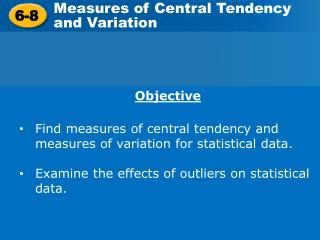DownloadDownload Presentation6-8

# 6-8

Télécharger la présentation## 6-8

- - - - - - - - - - - - - - - - - - - - - - - - - - - E N D - - - - - - - - - - - - - - - - - - - - - - - - - - -
##### Presentation Transcript

1. Measures of Central Tendency and Variation 6-8 • Objective • Find measures of central tendency and measures of variation for statistical data. • Examine the effects of outliers on statistical data.

2. Reading Math The symbol commonly used to represent the mean is x, or “x bar.” The symbol for standard deviation is the lowercase Greek letter sigma, σ. Small standard deviations indicate data clustered near the mean Large standard deviations indicate data is spread out

3. Finding Standard Deviation

4. Example 1: Finding the Mean and Standard Deviation Find the mean and standard deviation for the data set of the number of people getting on and off a bus for several stops. {6, 8, 7, 5, 9} Step 1 Find the mean. Step 2 Find the difference between the mean and each data value, and square it.

5. Example 1: Finding the Mean and Standard Deviation Find the mean and standard deviation for the data set of the number of people getting on and off a bus for several stops. {6, 8, 7, 5, 9} Step 1 Find the mean. Step 2 Find the difference between the mean and each data value, and square it. -1 1 0 -2 2 1 1 0 4 4

6. Example 1 Continued -1 1 0 -2 2 1 1 0 4 4 Step 3 Find the variance. Find the average of the last row of the table Step 4 Find the standard deviation. The standard deviation is the square root of the variance The mean is 7 people the standard deviation

7. Example 1 Continued -1 1 0 -2 2 1 1 0 4 4 Step 3 Find the variance. Find the average of the last row of the table Step 4 Find the standard deviation. The standard deviation is the square root of the variance The mean is 7 people the standard deviation

8. Example 2 Find the mean and standard deviation for the data set of the number of elevator stops for several rides. {0, 4, 2, 1, 6, 5} Step 1 Find the mean. Step 2 Find the difference between the mean and each data value, and square it.

9. Example 2 Find the mean and standard deviation for the data set of the number of elevator stops for several rides. {0, 4, 2, 1, 6, 5} Step 1 Find the mean. Step 2 Find the difference between the mean and each data value, and square it. -3 1 -1 -2 2 3 9 1 1 4 4 9

10. Example 2Continued -3 1 -1 -2 2 3 9 1 1 4 4 9 Step 3 Find the variance. Find the average of the last row of the table Step 4 Find the standard deviation. The standard deviation is the square root of the variance The mean is __, and the standard deviation is

11. Example 2Continued -3 1 -1 -2 2 3 9 1 1 4 4 9 Step 3 Find the variance. Find the average of the last row of the table Step 4 Find the standard deviation. The standard deviation is the square root of the variance The mean is 3, and the standard deviation is

12. Classwork/Homework 6-8Worksheet